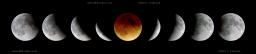# Determine 2937

Determine the first 14 members of the sequence if a15 = 225, d = -12

a14 =  237
a13 =  249
a12 =  261
a11 =  273
a10 =  285
a9 =  297
a8 =  309
a7 =  321
a6 =  333
a5 =  345
a4 =  357
a3 =  369
a2 =  381
a1 =  393

### Step-by-step explanation:Did you find an error or inaccuracy? Feel free to write us. Thank you!